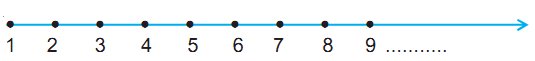# Natural Numbers

The counting numbers 1, 2, 3, ... constitute the system of natural numbers. These are the numbers which are used in day-to-day life.

There is no greatest natural number, for if 1 is added to any natural number, you get the next higher natural number, called its successor.

### Number Line

The natural numbers can be represented on a number line:### Operations of Natural Numbers

There are four-fundamental operations on natural numbers - addition, subtraction, multiplication and division. For example,

• 4 + 2 = 6, again a natural number
• 6 + 21 = 27, again a natural number
• 22 – 6 = 16, again a natural number, but 2 – 6 is not defined in natural numbers
• 4 × 3 = 12, again a natural number
• 12/2 = 6, again a natural number

Addition and multiplication of natural numbers again yield a natural number but subtraction and division of two natural numbers may or may not yield a natural number.

Two natural numbers can be added and multiplied in any order and the result obtained is always same. This does not hold for subtraction and division of natural numbers.